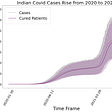# Introduction

Descriptive Statistics is creating a summary of data through quantitative measures like mean, median, etc. so as to get a better understanding of the data. It does not involve any generalization or inference beyond what is available. This means that descriptive statistics are just the representation of the data (sample) available and not based on any theory of probability.

# Measures of Central Tendency

There are 3 measures of central tendency:

1. Mean: It is also known as a simple average. It is the most common measure of central tendency. But it has a huge downside is it gets easily affected by outliers (abruptly high values present in data that have a high effect on the mean). We cannot make conclusions based on only means.
2. Median: It is the number at the central position in an ordered list if the length is an odd number and the average of the two center values if the length is even. We can clearly see that the median is not affected by the outliers. But, still using it alone will not help us reach a conclusion.
3. Mode: It is the value that occurs most often. It can be used for both numeric as well as categorical data. If the frequency of occurrence of all data is the same then we say that there is no mode. In the real-world application, we can consider 2 or 3 modes that are having the same value of occurrences.

None of the above Measures are the best. They do not provide enough information alone but together they can give us a good idea of the central tendency of the data.

# Measures of Asymmetry

Skewness: It is the measure of where the data is concentrated.

The skewness can be observed using the measures of central tendency. It is important as it tells us where our data affecting the entire results are situated.

Case 1: If Mean < Median Then the data is left-skewed.

Case 2: If Mean = Median = Mode Then the data is centrally skewed or in a normal distribution.

Case 3: If Mean > Median Then the data is right-skewed.

# Measures of Variability

There are many ways to measure Variability. Some main ways are:

1. Variance
2. Standard Deviation
3. Coefficient of Variation

In the field of Statistics, we have different formulas for sample data as well as population data. In population data, you are sure of the measures you are calculating as you know the entire data. But in sample data, every time you take the sample the measures will be different as sample data is an approximation of the population parameter. Hence we have different formulas for a sample as well as population data.

# Coefficient of Variation

It is the ratio of Standard Deviation to the mean. It is a better measurement tool for comparing multiple but similar datasets as it is a unitless quantity.

# Variance

Variance measures the dispersion of a set of data points around their mean.

# Standard Deviation

In real-world applications, the value of variance is very large and it is hard to compare. So, we take the square root of the variance and it is known as Standard Deviation. It is the most commonly used measure of variability for a single dataset.

# Measures of Relationship between variables

There are different ways to measure the relationship between variables but the most common are:

1. Covariance
2. Correlation coefficient

# Covariance

Covariance is the measure of the relationship between two variables. Unlike variance-covariance can be either positive, negative, or even zero.

Covariance gives us a sense of direction where the two variables are moving.

If the value of covariance is positive it means that the two variables move together. If the covariance is negative it means that the two variables move in opposite directions. If the value of covariance is zero then we can say that the two variables are independent of each other.

# Correlation Coefficient

Correlation adjusts covariance so that the relationship between the two variables becomes easy and intuitive to interpret. It is the ratio of covariance to the multiplication of standard deviations of individual variables.

The value of correlation coeff lies between -1 to 1 and is quite similar to coeff of variance.

--

--

--

## More from Dhruv Khanna

Data Science Enthusiast

Love podcasts or audiobooks? Learn on the go with our new app.

## New Encodings to Improve ClickHouse Efficiency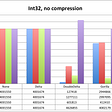## 6 Critical Dimensions of Data Quality## Linear Regression. Back to Basics.## What are data brokers and why should you care?## Announcing ODSC West 2020 Bootcamp Specialization Tracks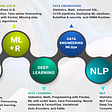## Q-Q Plots — A view from statistics perspective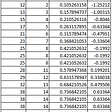## Data Storage Keeping Pace for AI and Deep Learning## State of Aadhaar initiative enters a new phase## Dhruv Khanna

Data Science Enthusiast

## Python vs R in Data Science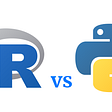## Linear Regression with real world application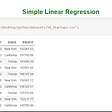## Things you should know about x̄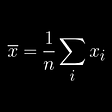## Mistakes In DataVisualization: A Short Practical Review (1)